Courses
Courses for Kids
Free study material
Free LIVE classes
More

# Equivalent Capacitance, Series, and Parallel Combination for JEELIVE
Join Vedantu’s FREE Mastercalss

## What is a Capacitor?

A capacitor is an electrical energy storage device made up of two conducting materials that are utilised in circuits. A battery charges capacitors in a basic circuit. When the capacitor is detached from the battery and attached to another component, the stored energy is discharged through that component and the rest of the circuit. While this discharge of energy allows the capacitor to operate as a "secondary battery" for the circuit, a capacitor that has once been discharged must be charged before it can be utilised again.

A basic capacitor is made up of two electrical conductors, and they are usually in the form of metallic plates or surfaces. They are separated by a dielectric medium. A foil, thin sheet, sintered metal bead, or electrolyte can all be used as conductors. The capacitor's charge capacity is increased by the non-conducting dielectric. Glass, ceramics, plastic film, paper, mica, air, and oxide layers are all common dielectric materials.

## Capacitance - Definition, Unit, and Formula

The capacitance of a capacitor refers to its ability to hold electrical energy, or charge (c). In standard units, capacitance is measured in Farads (F) or coulomb per volt.

The formula for the capacitance of a capacitor is given as

$C=\dfrac{Q}{V}$

Here Q is the amount of charge that the capacitor can hold and V is the potential difference across the plates of the capacitor.

When one capacitor's capacitance is greater than another's, it means Capacitor A can store more charge than Capacitor B. Although the capacitances of capacitors used for two different purposes may change, the energy storage and release procedure are the same for both. Capacitors are beneficial in situations where a speedy release of energy is required, such as powering the flash on a digital camera, because they can discharge stored energy considerably faster than a traditional battery.

## Equivalent Capacitance

The equivalent capacitance represents the sum of all capacitance values in a circuit, and it may be calculated by adding all individual capacitances in the circuit based on their relationships. Capacitors can be connected in two ways:

1. Parallel

2. Series

When capacitors are connected in series, the equivalent capacitance of the circuit increases, whereas when capacitors are connected in parallel, the equivalent capacitance of the circuit decreases. We will see the reason behind this and learn how to find equivalent capacitance in the next sections.

## Equivalent Capacitance in Parallel

The capacitors are said to be in a parallel combination when the two terminals of one capacitor are connected to each terminal of another capacitor. All the capacitors that are connected in parallel have the same voltage. This is the key to determining the combination's equivalent capacitance.

For calculating the equivalent capacitance, we will consider two capacitors having capacitance C1 and C2, respectively. Consider that one capacitor can hold Q1 charge and another can hold Q2 charge. The total charge stored by these capacitors is given as

$Q=Q_{1}+Q_{2}$

Consider the voltage across the capacitors to be V. Using the capacitance, we can write

\begin{align} &Q_{1}=C_{1} V \\ &Q_{2}=C_{2} V \end{align}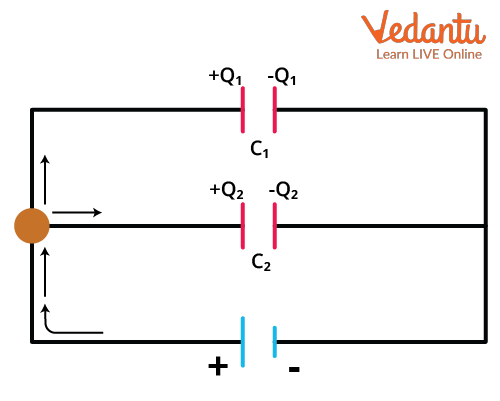Two Capacitors in Parallel

This means that

\begin{align} &C V=C_{1} V+C_{2} V \\ &C=C_{1}+C_{2} \end{align}

For an n number of capacitors in parallel, we can write the equivalent capacitance as

$C_{e q}=\sum_{1}^{n} C_{i}=C_{1}+C_{2}+C_{3}+\cdots$

This is the equivalent capacitance formula for capacitors connected in parallel.

## Equivalent Capacitance in Series

When one capacitor's terminal is connected end to end to the terminal of another capacitor, this is known as a series combination (much like railway coaches connected one after the other). In a series, each capacitor receives the same charge.

If the capacitors in the series connection are connected to a battery then each capacitor in the series connection will extract some part of the voltage of the battery. The total potential difference of the battery will be equal to the sum of the voltages extracted by each capacitor.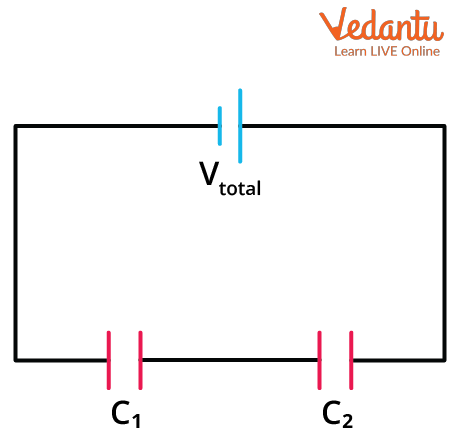Capacitors in Series Combination

Suppose we have two capacitors of capacitance C1 and C2, respectively. Let’s consider that C1 extracts V1  volts and C2 extracts V2, volts from the battery whose potential difference is V. So, we can write

$V=V_{1}+V_{2}$

Let each capacitor receive charge Q, so for both capacitors, we can write

$\dfrac{Q}{C}=\dfrac{Q}{C_{1}}+\dfrac{Q}{C_{2}}$

Here, C is the equivalent capacitance. We write the above equation using

$V=\dfrac{Q}{C}$

We can further simplify it as

$\dfrac{1}{C}=\dfrac{1}{C_{1}}+\dfrac{1}{C_{2}}$

For n number of capacitors connected in series the equivalent capacitance will be

$\dfrac{1}{C_{e q}}=\sum_{1}^{n} \dfrac{1}{C_{i}}=\dfrac{1}{C_{1}}+\dfrac{1}{C_{2}}+\dfrac{1}{C_{3}}+\cdots$

This is the equation for equivalent capacitance in series.

## Numerical Problems on Equivalent Capacitance

Example 1: Find the equivalent capacitance of the circuit given below. Also, find the charge on the equivalent capacitance if a potential difference of 5V is applied by the battery.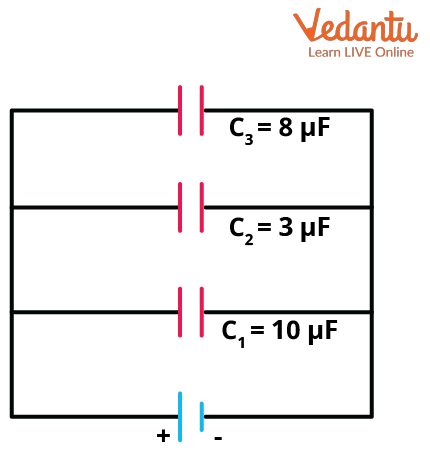Find Equivalent Capacitance

Solution: Since the capacitors are connected in parallel, the equivalent capacitance can be written as

\begin{align} &C=C_{1}+C_{2}+C_{3} \\ &C=10+3+8 \\ &C=21 \mu \mathrm{F} \end{align}

The charge on the equivalent capacitance will be given by

Q=CV

We have V=5 volts.

\begin{align} &Q=21 \times 10^{-6} \times 5 \\ &Q=105 \times 10^{-6} \\ &Q=0.105 \mathrm{mC} \end{align}

So the equivalent capacitance is 21$\mu F$ and the charge stored by the equivalent capacitance is 0.105mC.

Example 2:  Find the equivalent capacitance of the circuit given below.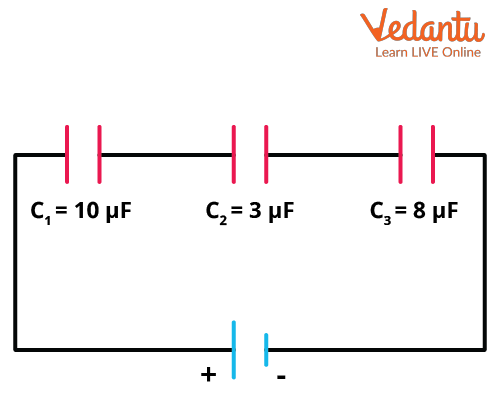Find Equivalent Capacitance

Solution: Since the capacitors are connected in series, their equivalent capacitance can be given by

\begin{align} &\dfrac{1}{C}=\dfrac{1}{C_{1}}+\dfrac{1}{C_{2}}+\dfrac{1}{C_{3}} \\ &\dfrac{1}{C}=\dfrac{1}{10}+\dfrac{1}{3}+\dfrac{1}{8} \\ &C \simeq \dfrac{1}{0.100+0.333+0.125} \\ &C \simeq 1.8 \mu \mathrm{F} \end{align}

So, the equivalent capacitance of the given circuit is approximately 1.8$\mu F$.

Example 3: From the circuit given below, find the charge on the 2μF capacitor.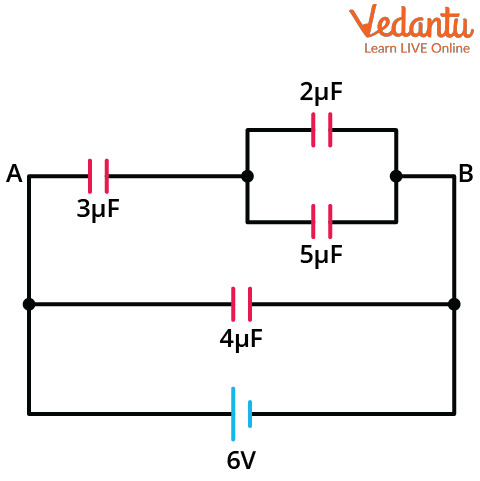Find Equivalent Capacitance

Solution: The potential across the points A and B will be 6V since they are connected in parallel with the battery. Now to find the equivalent capacitance between AB, we will use the fact that $2\mu F$ and $5\mu F$ capacitors are connected in parallel and this combination is connected in series with a 3μF capacitor. The equivalent capacitance of $2\mu F$ and $5\mu F$ will be

$2+5=7~\mu F$

Now, this combination is connected in series with a $3\mu F$ capacitor. So the equivalent capacitance between A and B will be

\begin{align} &\dfrac{1}{C_{A B}}=\dfrac{1}{3}+\dfrac{1}{7} \\ &C_{A B}=\dfrac{3 \cdot 7}{3+7} \\ &C_{A B}=\dfrac{21}{10} \\ &C_{A B}=2.1 \mu \mathrm{F} \end{align}

The total charge of the AB branch can be given as

\begin{align} &q=C_{A B} \times V \\ &q=2.1 \times 6 \\ &q=12.6 \mu \mathrm{C} \end{align}

The potential difference across the $3\mu F$ capacitor can be given as,

\begin{align} &V_{3}=\dfrac{q}{3} \\ &V_{3}=\dfrac{12.6}{3} \\ &V_{3}=4.2 \mathrm{~V} \end{align}

The potential difference across the parallel combination of $2\mu F$ and $5\mu F$ will then be

\begin{align} V_{p}=6-4.2 \\ V_{p}=1.8 \mathrm{~V} \end{align}

Since the potential difference in a parallel combination is the same for both capacitors, then the charge across the 2μF capacitor can be given as

\begin{align} &q_{2}=2 \times V_{p} \\ &q_{2}=2 \times 1.8 \\ &q_{2}=3.6 \mu \mathrm{C} \end{align}

So, the charge across the $2\mu F$ capacitor is $3.6\mu C$.

## Conclusion

The capacitor is a passive electrical device that is usually made up of two conductors, and it stores charges. It stores electric charges and then converts this charge into electrical energy when required. It functions a lot like a battery. The capacitance of a capacitor is basically a measure of the amount of charge that it can hold. Capacitance is equal to the ratio of charge and the voltage across the plates. The reciprocal of the series capacitance of the equivalent resistance is equal to the sum of the reciprocals of the individual capacitances. For a parallel connection, the equivalent capacitance is equal to the sum of the individual capacitances.

Last updated date: 18th Sep 2023
Total views: 127.8k
Views today: 2.27k

## FAQs on Equivalent Capacitance, Series, and Parallel Combination for JEE

1. What is the weightage of equivalent capacitance in JEE main?

Equivalent capacitance is an important topic from the exam point of view, and it is a part of Electrostatics. Electrostatics has about 10% weightage in JEE main. The capacitance and grouping of capacitors have a lot of application and circuit-based problems that may appear in any exam. Not just for JEE Main, this topic is also important for other engineering entrance exams. The series and parallel capacitance formulae must be remembered as they are used extensively to solve circuits.

2. What are some applications of capacitors?

Capacitors are electrical charge storage devices. They are a fundamental component of electronics and have a wide range of uses. Capacitors are most commonly used for energy storage. Power conditioning, signal coupling or decoupling, electronic noise filtering, and remote sensing are some other applications. Capacitors are utilised in a wide range of sectors and have become an essential component of daily life due to their numerous applications. Recently a lot of research is being done on supercapacitors and their applications.